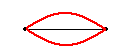# What will the wave velocity be in this string?

## Homework Statement

What will be the wave velocity, if a string of 100 cm length is in a 10 kg-wt tension forms 9 nodes when vibrated and becomes symphonic to a 50 Hz tuning fork. Given, Cross sectional area of string, $$A=4.95 mm^2$$ & density, $$d = 0.25 g/cc$$?

## Homework Equations

$$V=\sqrt{\dfrac{T}{m}}$$

m= mass per unit length

T = Tension in string

## The Attempt at a Solution

Velocity in a string
$$V=\sqrt{\dfrac{T}{m}}$$
Where,

Tension in string, $$T= 10\times9.8=98 kN$$
mass per unit length, $$m= \frac{v \times d}{L}=A\times d= 0.012225 kg/m$$
So,$$V = 89.534 m/s$$
.Now the problem is answer should be equal to 50 m/s . Is there any effect of tuning fork on the wave velocity of the string ?

Last edited:

kuruman
Homework Helper
Gold Member
2021 Award
Hi Zakiyah Afrin and welcome to PF.

Mass is density multiplied by volume, not density multiplied by cross sectional area. To find the volume, you need the length of the string. How can you find an expression for that?

•Zakiyah Afrin
I mean mass per unit length i.e $$m= \frac{v \times d}{L}=A\times d$$

kuruman
Homework Helper
Gold Member
2021 Award
And what do you get with this correction?

On Edit: Actually, yes m = A d is correct for "mass per unit length". I interpreted "m" as total mass. What do you get for this in units of kilograms per meter?

Last edited:
•Zakiyah Afrin
And what do you get with this correction?
no I calculated in right way. May be there Is something with the frequency

kuruman
Homework Helper
Gold Member
2021 Award
You appear to have made some mistakes. When you say
##T= 10\times9.8=98 kN## this is not correct. ##T= 10\times9.8=98 N##. ##1~kN=1000~N##. That's minor. You seem to have used 98 N in your calculation so no harm done.
You seem to have made some error in your calculation for ##d##. When you convert 0.25 g/cc, remember that 1 g = 10-3 kg and 1 cc = 10-6 m3.
Now for the important part. You are given the tension ##T##, and enough information to find the linear density ##\mu## (I prefer to use ##m## for total mass). Then it should be a straightforward calculation to find the velocity using ##v=\sqrt{T/\mu}## which is what you attempted. The problem is that if you put in the correct values for ##T## and ##\mu##, you do not get 50 m/s.

However, if you completely ignore the tension and the linear density and assume that "becomes symphonic to a 50 Hz tuning fork" means that the vibrating frequency of the string is a harmonic of 50 Hz (which one?) you can get 50 Hz (how?)

This problem bothers me because the sets of information it provides appear to be internally inconsistent.

•Zakiyah Afrin
And what do you get with this correction?

On Edit: Actually, yes m = A d is correct for "mass per unit length". I interpreted "m" as total mass. What do you get for this in units of kilograms per meter?
as I mentioned 0.01225

kuruman
Homework Helper
Gold Member
2021 Award
as I mentioned 0.01225
Repeating the number, does not help if you do not show what went in the calculation. Your number is 10 times what it should be. Also, be sure to include units every time you quote a number. It's a good habit.•Zakiyah Afrin
Sorry, I did lot of mistakes.
F=98 N
μ=0.001225 kg/m
v= 283.13 m/s
T= 98 N
but frequency for this becomes
141.57 Hz

kuruman
Homework Helper
Gold Member
2021 Award
Sorry, I did lot of mistakes.
F=98 N
μ=0.001225 kg/m
v= 283.13 m/s
T= 98 N
but frequency for this becomes
141.57 Hz
Yes, that's what I got when I used ##v=\sqrt{T/\mu}##. Please read post #6. You can find v = 50 m/s if you use v = λ f, but you need to find λ and f separately. Hint: f is not 50 Hz but a harmonic of 50 Hz.

•Zakiyah Afrin
Yes, that's what I got when I used ##v=\sqrt{T/\mu}##. Please read post #6. You can find v = 50 m/s if you use v = λ f, but you need to find λ and f separately. Hint: f is not 50 Hz but a harmonic of 50 Hz.
since, no. of nodes = 9
so it will be 8th harmonic I guess n= 8
f = n f0=8*50=400 Hz
λ = 2*L/n=1/4m
$$v=f λ=400*1/4=100 ms^{-1}$$
but not 50 m\s

kuruman
Homework Helper
Gold Member
2021 Award
How do you get harmonics from nodes? How many nodes does the fundamental ##n=1## have? Draw a picture if you must.

•Zakiyah Afrin
How do you get harmonics from nodes? How many nodes does the fundamental ##n=1## have? Draw a picture if you must.for n= 1 number of nodes = 2

#### Attachments

kuruman
Homework Helper
Gold Member
2021 Award
I have a different counting scheme that excludes the ends, but that's OK as long as you know what you mean. In that case I agree with calculation of 100 m/s in post #11. Unless I misunderstand what "becomes symphonic" means, I don't see how one gets 50 m/s.

Then, there is a question fault right ?

kuruman